## A body of mass 2 kg moves in a (counterclockwise) circular path of radius 3 meters, making one revolution every 5 seconds. You may assume th

Question

A body of mass 2 kg moves in a (counterclockwise) circular path of radius 3 meters, making one revolution every 5 seconds. You may assume the circle is in the xy-plane, and so you may ignore the third component.
A. Compute the centripetal force acting on the body.
B. Compute the magnitude of that force.

in progress 0
2 months 2021-07-24T01:13:59+00:00 1 Answers 5 views 0

a)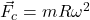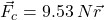b)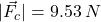Step-by-step explanation:

a) The centripetal force equation is: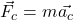Now, we know that the body makes one revolution every 5 seconds, so we can find the angular velocity: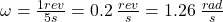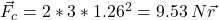The centripetal force is a vector in the radius direction.

b) The magnitude of that force will be: